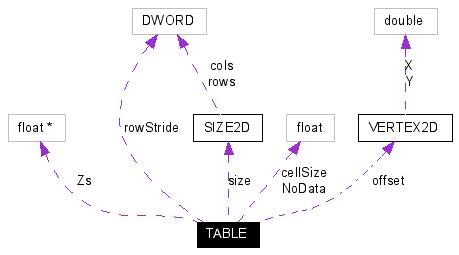﻿ TABLE structure

TABLE structure

Declared in: GeoTransformers.h

Grid of float Z values. Usually represents a Digital Elevation Model (DEM).

[C / C++]

typedef struct {

float NoData; // value of Zs to be ignored (usually -9999)

float * Zs; // grid Z values

float cellSize; // grid's scale

VERTEX2D offset; // coordinates of the upper-left corner of the grid

SIZE2D size; // grid size

DWORD rowStride; // offset between consecutive rows (used to process regions)

} TABLE;

Collaboration diagram:Collaboration graph for TABLE structure

Members

NoData

Value of elevations to be ignored by the transformation (usually -9999). It is used to mark holes in the grid that shouldn't be changed.

Zs

Encapsulated array of elevations. The size of this one-dimensional array can be determined by multiplying the number of columns with the number of rows specified by size member. The first element of the array represents the upper-left corner value of the grid. The second entry belongs to the same row as the first (if more than one column).

cellSize

Scale of the grid. It is used to increment X coordinate and decrement Y coordinate when parsing the grid from its offset.

offset

Grid's origin, representing the upper-left corner of the grid. The value of the first element in the encapsulated array represents the elevation of this (X,Y) pair.

size

Grid dimensions, described as number of columns and rows.

rowStride

The row address stride. It is used to process only regions of a larger grid. When its value is zero, then there's no shift to be applied to jump to a consecutive row. Otherwise, its value represents the number of columns in the grid of which region we were given. It means that from the address of the beginning of a row to the beginning of the next, there are exactly rowStride * sizeof(float) bytes.

Remarks

Very few GeoTransformers will process this type of data. When a transformation also changes X and Y coordinates, most probably the grid should be re-interpolated.

Requirements

 Platform 32 bit Windows OS Environment ANSI C / C++ Standard compliant

Related Topics

Referenced By: GeoTransData::GTD

References: VERTEX2D, SIZE2D